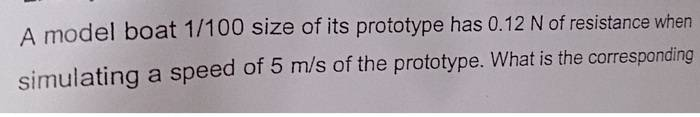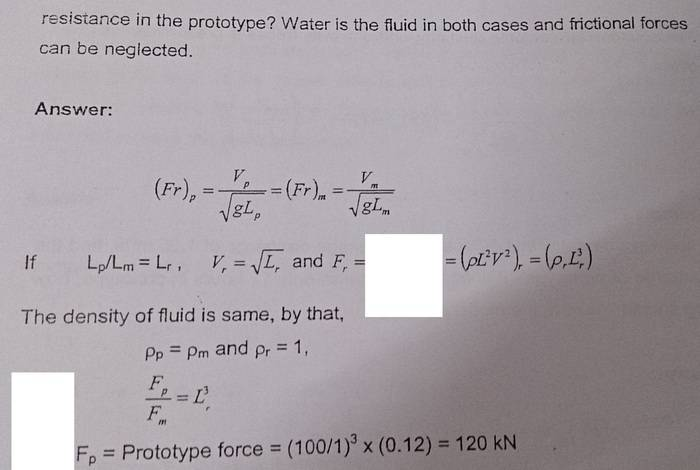# Formula of forces

foo9008

## Homework Statement

from the notes , the author stated that force has the formula of ρ(L^2)(v^2) and also = ρ(L^3)
i think there's something wrong with the ρ(L^3)## The Attempt at a Solution

IMO , ρ(L^2)(v^2) can also be written as ρ(L^4)(T^2) , so Force is proportional to L^4 , am i right . ? IMO, the prototype force should be [ (100^4) ] x 0.12 N

correct me if i am wrong ...

tommyxu3
I'm not sure, but in your picture, one is ##\rho## and the other is ##\rho_r.## Maybe there is detailed definitions in the problem?

foo9008
I'm not sure, but in your picture, one is ##\rho## and the other is ##\rho_r.## Maybe there is detailed definitions in the problem?
rho r is actually r , it's the pi buckingham theorem

Homework Helper
Gold Member
ρ(L^2)(v^2) can also be written as ρ(L^4)(T^2) , so Force is proportional to L^4
I guess you mean ρ(L^4)(T^-2), but either way it does not follow. The whole point of figuring out these dimensionless expressions is that you cannot change the scale of L and assume T fixed, etc. There are physical constants in the system, like viscosity, which impose a relationship between the fundamental dimensions. Changing L keeping T etc. fixed will therefore change the viscosity.
Similarly, density fixes a relationship between M and L.

foo9008
I guess you mean ρ(L^4)(T^-2), but either way it does not follow. The whole point of figuring out these dimensionless expressions is that you cannot change the scale of L and assume T fixed, etc. There are physical constants in the system, like viscosity, which impose a relationship between the fundamental dimensions. Changing L keeping T etc. fixed will therefore change the viscosity.
Similarly, density fixes a relationship between M and L.
Then, what is the correct formula of force in this question?

foo9008
I guess you mean ρ(L^4)(T^-2), but either way it does not follow. The whole point of figuring out these dimensionless expressions is that you cannot change the scale of L and assume T fixed, etc. There are physical constants in the system, like viscosity, which impose a relationship between the fundamental dimensions. Changing L keeping T etc. fixed will therefore change the viscosity.
Similarly, density fixes a relationship between M and L.
so , the author is correct ? it is (rho)(L^2)(L ) , where (v^2) = L ??

Homework Helper
Gold Member
so , the author is correct ? it is (rho)(L^2)(L ) , where (v^2) = L ??
I'm hampered by not knowing what Fr stands for in the first line.
I presume F=ρL2V2 comes from some earlier work.
If we accept both of those equations, the rest follows.

foo9008
I'm hampered by not knowing what Fr stands for in the first line.
I presume F=ρL2V2 comes from some earlier work.
If we accept both of those equations, the rest follows.
So, the authors working is correct??